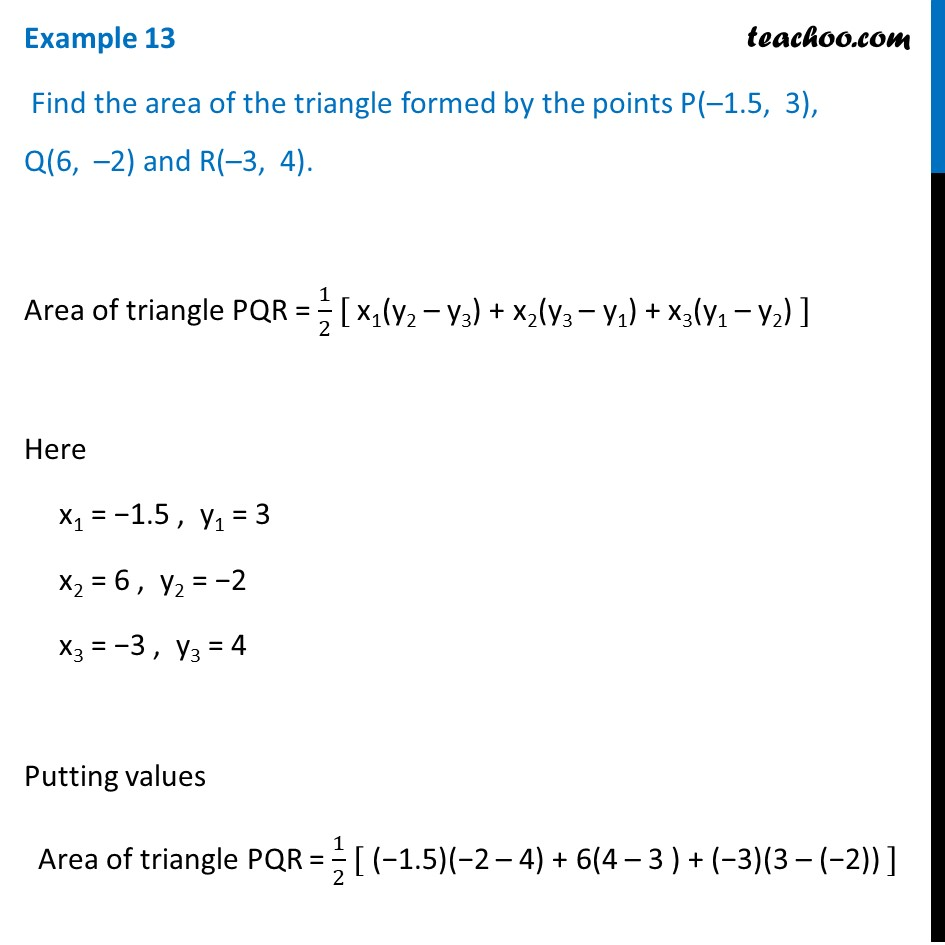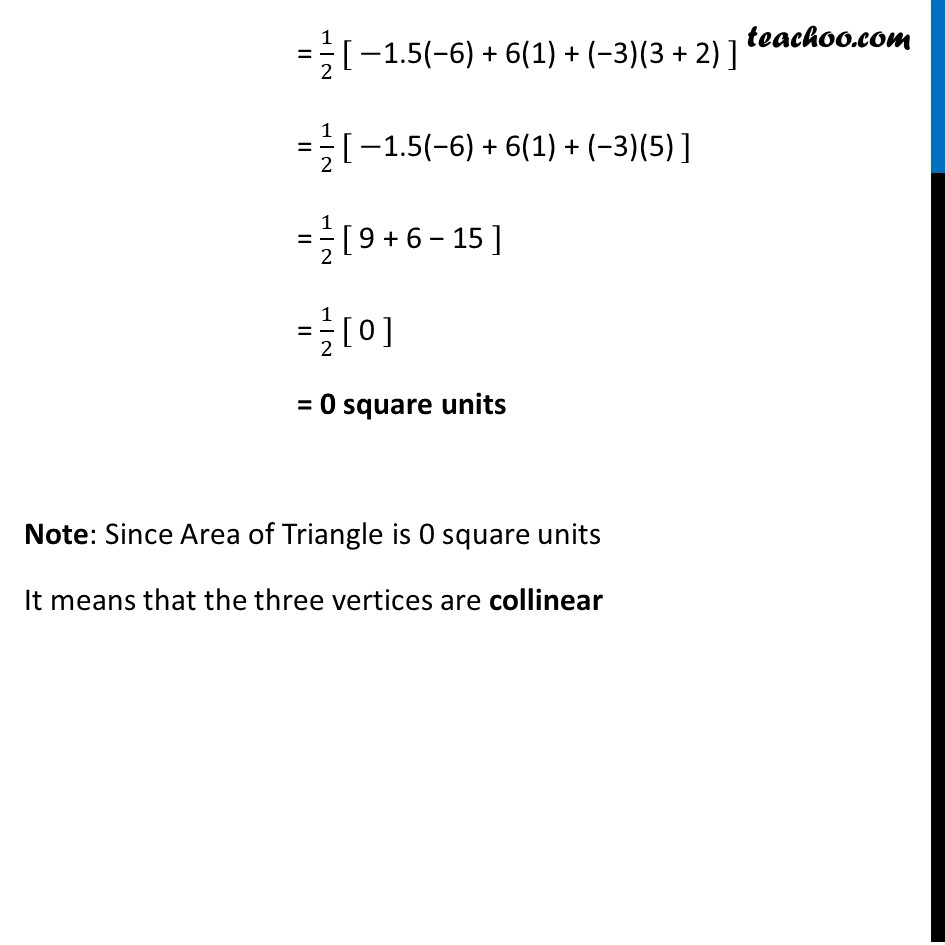Examples

Chapter 7 Class 10 Coordinate Geometry
Serial order wiseLearn in your speed, with individual attention - Teachoo Maths 1-on-1 Class

### Transcript

Question 3 Find the area of the triangle formed by the points P(–1.5, 3), Q(6, –2) and R(–3, 4). Area of triangle PQR = 1/2 [ x1(y2 – y3) + x2(y3 – y1) + x3(y1 – y2) ] Here x1 = −1.5 , y1 = 3 x2 = 6 , y2 = −2 x3 = −3 , y3 = 4 Putting values Area of triangle PQR = 1/2 [ (−1.5)(−2 – 4) + 6(4 – 3 ) + (−3)(3 – (−2)) ] = 1/2 [ −1.5(−6) + 6(1) + (−3)(3 + 2) ] = 1/2 [ −1.5(−6) + 6(1) + (−3)(5) ] = 1/2 [ 9 + 6 − 15 ] = 1/2 [ 0 ] = 0 square units Note: Since Area of Triangle is 0 square units It means that the three vertices are collinear